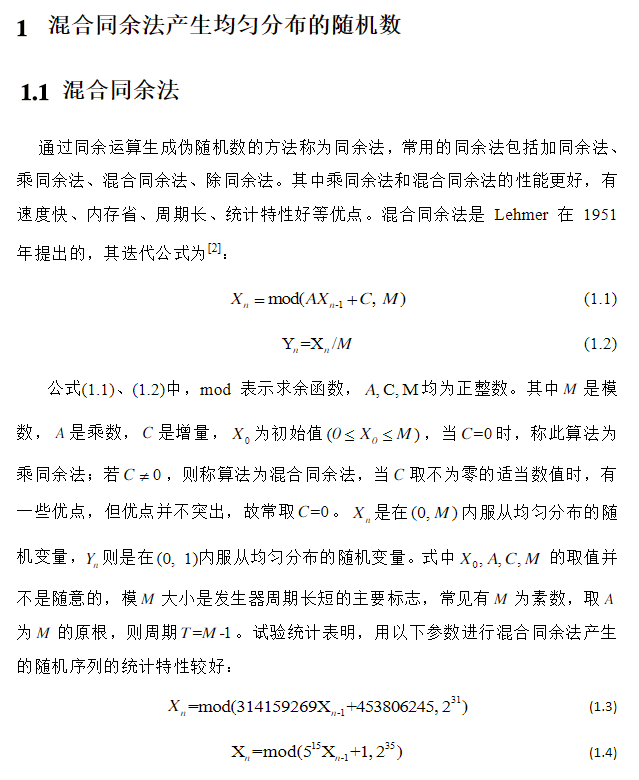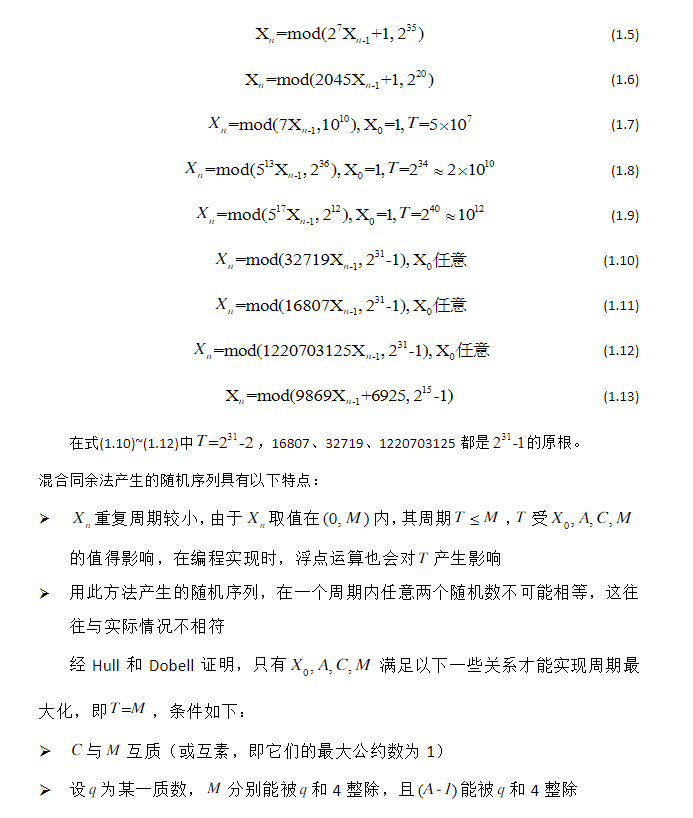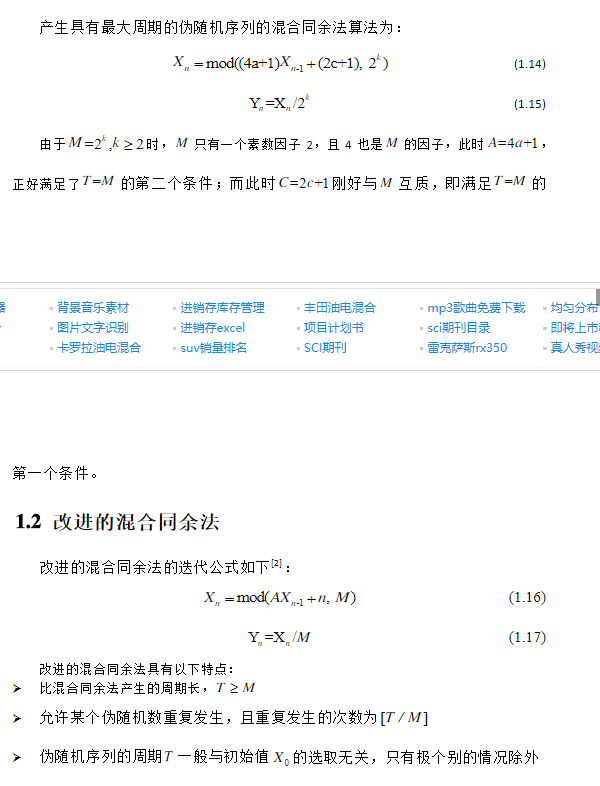• 通过对混合同余法产生随机序列的原理研究，本文提出了一种利用FPGA产生高斯白噪声的方法。该方法在PC主控端的控制下，采用ROM查找表的方式实现信号的产生。在混合同余法的原理基础上，设计利用 MATLAB和QuartusⅡ...
• 混合同余法怎么产生均匀随机数。 包括算法的讲解，和改进。
• 混合同余法产生均匀分布随机数产生方法详细总结及其相应的改进方法。。。。。。
• 混合同余法产生随机数和M序列产生方法（算例及matlab程序），参考文献《过程辨识》，方崇智，肖德云，清华大学出版社
•展开全文• 在用计算机编制程序时，经常需要用到随机数，尤其在仿真等领域，更对随机数的产生提出了较高的要求，仅仅使用 C 语言类库中的随机函数已难以胜任相应的工作。现实中，用投色子计数的方法产生真正的随机数，但电脑若...
在用计算机编制程序时，经常需要用到随机数，尤其在仿真等领域，更对随机数的产生提出了较高的要求，仅仅使用 C 语言类库中的随机函数已难以胜任相应的工作。现实中，用投色子计数的方法产生真正的随机数，但电脑若也这样做，将会占用大量内存；虽然用噪声发生器或放射性物质也可产生真正的随机数，但操作不可重复。而用数学方法产生随机数则最适合计算机，这就是周期有限，易重复的“伪随机数”。
（注：这里生成的随机数所处的分布为 0-1 区间上的均匀分布。不是 0-1 区间怎么办？ 除以 (high-low), 再加上 low 就可以完成任务。我们需要的随机数序列应具有非退化性，周期长，相关系数小等优点。）

1、迭代取中法：
这里在迭代取中法中介绍平方取中法 , 其迭代式如下 :
Xn+1=(Xn^2/10^s)(mod 10^2s)
Rn+1=Xn+1/10^2s
其中，Xn+1是迭代算子，而 Rn+1 则是每次需要产生的随机数。
第一个式子表示的是将 Xn 平方后右移 s 位，并截右端的 2s 位。
而第二个式子则是将截尾后的数字再压缩 2s 倍，显然 :0=<Rn+1<=1。
迭代取中法有一个显著的不良特性就是它比较容易退化成 0。

实现代码（C语言）：
#include <stdio.h>
#include <math.h>
#define S 2

float Xn=12345;  //Seed & Iter
float Rn;        //Return Val

void InitSeed(float inX0)
{
Xn=inX0;
}

float MyRnd()
{
//implementation: Xn+1=(Xn^2/10^S)(mod 10^2S)
Xn=(int)fmod((Xn*Xn/pow(10,S)),pow(10,2*S));//here can's use %

//implementation: Rn+1=Xn+1/10^2S
Rn=Xn/pow(10,2*S);

return Rn;
}

/*测试主程序，注意，本文只列举一次测试主程序，以下不再重复*/
int main()
{
int i;
FILE * debugFile;
if((debugFile=fopen("outputData.txt","w"))==NULL)
{
fprintf(stderr,"open file error!");
return -1;
}
printf("\n");
for(i=0;i<100;i++)
{
tempRnd=MyRnd();
fprintf(stdout,"%f ",tempRnd);
fprintf(debugFile,"%f ",tempRnd);
}
getchar();
return 0;
}

前一百个测试生成的随机数序列（明显可见其容易退化为0）：
0.399000 0.920100 0.658400 0.349000 0.180100 0.243600 0.934000 0.235600 0.550700 0.327000 0.692900 0.011000 0.012100 0.014600 0.021300 0.045300 0.205200 0.210700
0.439400 0.307200 0.437100 0.105600 0.115100 0.324800 0.549500 0.195000 0.802500 0.400600 0.048000 0.230400 0.308400 0.511000 0.112100 0.256600 0.584300 0.140600 0.976800 0.413800 0.123000 0.512900 0.306600 0.400300 0.024000 0.057600 0.331700 0.002400 0.000500
0.000000 0.000000 0.000000 0.000000 0.000000 0.000000 0.000000 0.000000 0.000000 0.000000 0.000000 0.000000 0.000000 0.000000 0.000000 0.000000 0.000000 0.000000 0.000000 0.000000 0.000000 0.000000 0.000000 0.000000 0.000000 0.000000 0.000000 0.000000 0.000000
0.000000 0.000000 0.000000 0.000000 0.000000 0.000000 0.000000 0.000000 0.000000 0.000000 0.000000 0.000000 0.000000 0.000000 0.000000 0.000000 0.000000 0.000000 0.000000 0.000000 0.000000 0.000000 0.000000 0.000000

2、乘同余法：
乘同余法的迭代式如下：
Xn+1=Lamda*Xn(mod M)
Rn+1=Xn/M
当然，这里的参数选取是有一定理论基础的，否则所产生的随机数的周期将较小，相关性会较大。经过前人检验的两组性能较好的素数取模乘同余法迭代式的系数为:
Lamda=5^5,M=2^35-31
Lamda=7^5,M=2^31-1
实现代码（C语言）关键部分：

double long  M;//请注意，这里一定要用到double long,否则计算2^32会溢出
float MyRnd()
{
Xn=fmod(Lamda*Xn,M);//here can's use %
Rn=Xn/M;
return Rn;
}

另外初始化段应有：
Lamda=pow(5,5);
M=pow(2,35)-31;

前三百个测试生成的随机数序列（可见该随机数生成方法所生成的随机序列比较符合0-1上的均匀分布,不过在某些数据段还有些起伏）：图1：  乘同余法生成的300随机数的产生序列图图2: 乘同余法生成的300随机数的分布情况

3、混合同余法：
混合同余法是加同余法和乘同余法的混合形式,其迭代式如下:
Xn+1=(Lamda*Xn+Miu)%M
Rn+1=Xn/M
经前人研究表明，在M=2^q的条件下，参数lamda,miu,X0按如下选取，周期较大，概率统计特性好:
Lamda=2^c+1,c取q/2附近的数
Miu=(1/2+sqrt(3))/M
X0为任意非负整数

实现代码（C语言）关键部分：
float MyRnd()
{
Xn=fmod(Lamda*Xn+Miu,M);
Rn=Xn/M;
return Rn;
}

另外初始化段应有：
M=pow(2,32);
Lamda=pow(2,16)+1;
Miu=(0.5+sqrt(3)/6)/M;

前三百个测试生成的随机数序列（可见该种随机数生成方法已相当接近0-1上的均匀分布。但在图3中可以看出它的一个致命的弱点，那就是随机数的生成在某一周期内成线性增长的趋势，显然，在大多数场合，这种极富“规律”型的随机数是不应当使用的。）：图3: 乘同余法生成的300随机数的产生序列图图4: 乘同余法生成的300随机数的分布情况


展开全文float 测试 语言 file c
• 随机数就是随机产生的数-_-||，分为两种：真随机数(random number)和伪随机数(pseudo-random number)。自然界由很多复杂因素产生的现象量化后的数（比如掷骰子）是真正随机的，就是真随机数。 ...
    随机数就是随机产生的数-_-||，分为两种：真随机数(random number)和伪随机数(pseudo-random number)。自然界由很多复杂因素产生的现象量化后的数（比如掷骰子）是真正随机的，就是真随机数。
一般意义上的电子计算机是确定系统，常规方法无法产生真随机数，而且真随机数的统计指标（原点矩、方差等）不一定好，且无法“重现”，故计算机常用伪随机数，又称为伪随机序列（pseudo-random sequence）。

/***************************加乘混合同余法****************************/

伪随机序列生成法有很多种，有取中法、移位法、同余法，近年来还有Gold序列（用于LTE系统加密）等等。
一般来说，伪随机序列常用[0,1]上的均匀分布，需要其他分布时，可以用概率积分变换快速地转换为其他分布
其中，生成算法比较简单，性能也比较高的算法是同余法中的加乘混合同余法，其公式表示为：其中C和 λ为常数，且C不为0， λ不为1（C=0是乘法同余法，λ=1是加法同余法）。
由于要得到[0,1]上的序列，于是以下不加证明（其实是我没找到，求高手给证明）地给出随机序列周期达到M的充要条件为：
1 )c 与M 互素;
2 ) 对每一个M 的素因子p ,λ - 1为p 的倍数;
3 ) 若M 是4 的倍数,那么λ- 1 是4 的倍数.
由以上条件易知，在2 进制计算机上,若,应取λ =4q + 1 ,c = 2 a + 1 ,为任意非负整数,其中q,a为正整数.
它的一个致命的弱点，那就是随机数的生成在某一周期内成线性增长的趋势，显然，在大多数场合，这种极富“规律”型的随机数是不应当使用的。

/****************随机序列质量的衡量指标***************************/
1、均匀性
比如以0.5为界，左右两边应几乎各占一半
2、覆盖性
对模拟需要的值，应几乎全部取到，不应有遗漏
3、循环性
不论序列有多长，应构成一个首尾相连的闭环序列
4、非循环性
这个意思是说“循环要足够长”，之前说循环性是指“能回来”，这里指“不能还没模拟完就回来”
5、独立性
随机序列应只取决于随机生成算法
6、检验性
随机函数要能经得起各种统计检验
7、系统开销要小
这个是大多数程序要求的，时间复杂度和空间复杂度要尽量低。
/*************蒙特卡罗方法*************************************/
Monte Carlo 方法的要点是:对要解决的数值计算问题,构造适当的概率模型,使要得到的解正好重合于概率模型中随机变量的概率分布或数字特征,其后在计算机上用伪随机数列对随机变量进行模拟得到一个大子样的观测数据,进行统计整理以后,给出问题的一个近似估计. 因此,Monte Carlo方法是双重近似,一是将数值计算问题用概率模型作近似,二是在计算机上用伪随机数作近似抽样值进行统计整理作出一些估计.
设g ( x ) 是[0 ,1 ] 上的连续函数, 且0 ≤ g ( x )≤1 . 考虑定定积分的值，那么由y=g(x)、y=0、x=0、x=1围成的图形面积就是S，如果在单位正方形(0,0,1,1)内均匀地投点(x , y ) ,则该随机点落入曲线y = g ( x ) 下阴影的概率：向正方形0 ≤x ≤1 ,0 ≤y ≤1 内均匀投点   ,ξi ,ηi 是相互独立的均匀随机数列,第i 次试验成功,即 落入A 中,也就是满足ηi ≤g (ξi ) ,若每次成功的概率为p ,进行n 次试验成功了k 次,则由大数定理知即n 充分大时, k/ n 依概率收敛到p . 由于p = s ,因此常取s ≈ k/ n .
于是，一个伪随机序列的质量可以用蒙特卡罗方法验证

/*****************翠花，上代码*********************************/
我们取
$M=2^30=1073741824$
$\lambda=129$

C=129
写的比较难看，大家凑合看一下吧……C++代码

1 #include <iostream> 2  #define M 1073741824 3  #define K 129 4  #define C 17 5  #define N 100000 6  const int n=3; 7  using namespace std; 8  long long t; 9 10  /**********加乘混合同余法**************/11  double rand(){12     double x;13     t=(K*t+C)%M;//t=Kt+c (mod M)14      x=double(t)/M;15     return x;16 }17 18  /*************乘方计算****************/19  long double power(long double x,long double n){20     long double y=x;21     for(int i=1;i<n;i++)22         y*=x;23     return y;24 }25 26  /*********** 蒙特卡罗积分法 *********/27  long double integrate(long double x[],long double y[]){28     long double s;29     long k=0;30     for(int i=0;i<N;i++){31         if(y[i]<=power(x[i],n))32             k++;33     }34     s=(long double)k/N;35     return s;36 }37 38  int main(){39     long double x[N],y[N],s=0.0,s2=0.0,d,e;40     int i;41     t=124;42     for(i=0;i<N;i++){43         x[i]=rand();44         s=s+x[i];//累加45         cout<<x[i]<<endl;46     }47     t=54;48     for(i=0;i<N;i++){49         y[i]=rand();50     }51     e=s/N;//平均数52     for(i=0;i<N;i++){53         s2+=(x[i]-e)*(x[i]-e);54         d=s2/(N-1);//方差 dafdq55     }56     cout<<"The average is "<<e57         <<",which is 0.5 theoretically"<<endl<<endl;58     cout<<"The mean square deviation is "<<d59         <<",which is 0.08333333 theoretically"<<endl<<endl;60     cout<<"Intgreate resault is "<<integrate(x,y)61         <<",which is "<<(double)1/(n+1)<< " theoretically"<<endl<<endl;62     return 0;63 }

给结果（序列太长了，略去）
The average is 0.500178,which is 0.5 theoreticallyThe mean square deviation is 0.0833717,which is 0.08333333 theoreticallyIntegreate result is 0.24989,which is 0.25 theoretically

%%%%%%%%%%%%Matlab程序%%%%%%%%%%%%%%%%%%%%%

用matlab测试的时候发现，先前选取的λ=65537并不好，在N=1000时，产生的序列是这样的一块一块的很难看，
把N降到一百非常明显的线性，这个缺点很大了！
所以我换了参数，并且与matlab自带的rand()进行了比较

clear;M=2^32;k=129;C=53;x(1)=63343;r(1)=63343/M;n=1000;m=rand(n,1);for i=2:n        x(i)=mod(k*x(i-1)+C,M);        r(i)=x(i)/M;endi=1:n;subplot(311)plot(i,r,'-r')subplot(312)plot(i,m,'-g');b=fft(r);c=abs(b);c(1)=0;                %0点有bugsubplot(313)plot(c);其中绿色的是matlab自带函数生成的序列，蓝色的是频谱图
*参考文献
 郭继展 过勇 苏辉 . 程序算法与技巧精选 ，机械工业出版社，2008
郑　列, 宋正义 伪随机数生成算法及比较 ，湖北工业大学学报，2008 年10 月

转载于:https://www.cnblogs.com/justin-wong/archive/2010/01/30/1660226.html
展开全文• 在很多计算机仿真算法及数字信号处理算法中，经常会用到随机数序列，比如进行蒙特卡洛仿真时就会用到。而且产生的随机数序列质量会对仿真结果产生比较大的影响，因此如何在仿真中产生随机数也是一个值得研究的课题。...
        在很多计算机仿真算法及数字信号处理算法中，经常会用到随机数序列，比如进行蒙特卡洛仿真时就会用到。而且产生的随机数序列质量会对仿真结果产生比较大的影响，因此如何在仿真中产生随机数也是一个值得研究的课题。事实上，通过计算机编程不可能实现真正的随机序列，因为产生的序列达到一定的长度后，会出现重复序列，使用的随机序列长度不应大于一个周期。这种随机数只能称作伪随机数。本文介绍常用的伪随机数发生器-迭代取中法，线性同余法和混合同余法在scilab下的实现。
迭代取中法，乘同余法及混合同余法的迭代公式如下：三种方法中，第二个公式均是为了得到[0,1]上的值，真正取随机迭代作用的是第一个式子。三种不同的方法各有特点。
1）迭代取中法容易退化成0。输入参数主要有种子，随机数长度，其scilab代码为:
function x=rand1(r0,n,s)
r=zeros(1,n+1);
x=zeros(1,n);
r(1)=r0;
for i=2:n+1
r(i)=pmodulo((r(i-1)^2)/10^s,10^(2*s)); //迭代
x(i-1)=r(i)/10^(2*s);                                   //取值范围为[0,1]
end
endfunction

function testofrand1
x=rand1(4532,500,2);               //产生500个数据，种子为4532,，S=2
n=1:size(x,2);
clf();
subplot(211);
plot2d(n,x,style=2);                    //画图
subplot(212);
histplot(10,x,style=3,normalization=%t); //画结果直方图，分为10类并归一化
endfunction

运行后，可以得到如下的结果可以看出，随着迭代的进行，350个数据之后基本上已经退化为0了，这也可以从直方图中看出来，前面的序列均匀性还是比较好的。
2）乘同余的周期与lambda和M密切相关，根据查到资料，有两组会效果比较好: Lambda=5^5,M=2^35-31 Lambda=7^5,M=2^31-1
其实现及测试代码如下：
function x=rand2(r0,n,lambda,M)   r=zeros(1,n+1);   x=zeros(1,n);   r(1)=r0;   for i=2:n+1     r(i)=pmodulo(lambda*r(i-1),M);     x(i-1)=r(i)/M;   end endfunction
function testofrand2   x=rand2(5.0,500,5^5,2^35-31);   clf();   subplot(211);   plot2d(x);   subplot(212);   histplot(10,x,style=2); endfunction
当Lambda=5^5,M=2^35-31，且序列长度为500时，效果如下当Lambda=5^5,M=2^35-31，且序列长度为1000时，效果如下可以看出均匀分布性还是比较好的，只是在个别段上会高一些，而且周期比迭代取中法长多了
3）混合同余法在如下条件下效果会比较好： M=2^q Lambda=2^c+1,c取q/2附近的值 Mu=(1/2+sqrt(3))/M X0为任意非负整数
其scilab实现及测试代码如下：
function x=rand3(r0,n,lambda,Mu,M)   r=zeros(1,n+1);   r(1)=r0;   x=zeros(1,n);   for i=2:n+1     r(i)=pmodulo(lambda*r(i-1)+Mu,M);     x(i-1)=r(i)/M;   end endfunction function testofrand3   M=2^10;   lambda=2^4+1;   Mu=(1/2+sqrt(3))/M;   x=rand3(5,10000,lambda,Mu,M);   clf();   subplot(211);   plot2d(x);   subplot(212);   histplot(10,x,style=2); endfunction
生成长度为10000的随机序列效果如下：可以看出，整体效果都很好，没有出现退化现象，有资料上说在某个周期内会出现线性增长，可是在这个结果中没有出现。
展开全文lambda function plot 算法 测试
• 现在的随机函数发生器大都采用...所谓线性同余法（又叫混合同余法），就是这样的一个公式：X[i+1]=(A*X[i]+C) mod M；经前人研究表明，在M=2^q的条件下，参数A，C，X按如下选取，周期较大，概率统计特性好:A=2^b+1=c 算法
• 现在的随机函数发生器...所谓线性同余法（又叫混合同余法），就是这样的一个公式：X[i+1]=(A*X[i]+C) mod M； 经前人研究表明，在M=2^q的条件下，参数A，C，X按如下选取，周期较大，概率统计特性好: A=2^b+1=2^
• 用java语言产生符合泊松分布的随机数...同余法是Lehmer于1951年提出来的此方法是利用数论中的同余运算原理来产生随机数有线性同余法非线性同余法等其中线性同余法法又分为加同余法乘同余法以及混合同余法同余法是现在发
• Matlab随机数生成的常用算法实现（用平方取中法产生随机数列 、用混合同余法产生随机数列 、用乘同余法1产生随机数列 、产生柯西分布的随机数列 、产生正态分布的随机数列 、产生贝努里-高斯分布的随机数列 ）
• 使用现代优化理论中的混合同余法，用C语言产生服从标准正态分布的随机数。
• 混合同余法生成随机数 C语言生成离散分布的原理及程序实现 两点分布和二项分布 C语言生成连续型随机变量 以正态分布为例
• 在音频中有时候用白噪声作为测试信号，下面是一个具体的简单白噪声产生算法。 算法参考文献：http://wenku.baidu.com/view/a643788dec3a87c24028c453 下面Code的算法使用的是“线性混合同余法”。
• 首先，由给定的初值x0，用混合同余法： {xi=(a∗xi−1+c)modMyi=xi/M \begin{cases} x_i=(a*x_{i-1}+c) mod M \\ y_i=x_i/M \end{cases} {xi​=(a∗xi−1​+c)modMyi​=xi​/M​ 产生（0, 1）区间上的随机数yi。 ...
• 一:试验原理。 （1）产生区间（0,1）上均匀分布的伪随机数（Z ~U (0,1)）。... 混合同余法：  由递推式  Y(1)=1;  Y(n+1)=(314159269Y(n)+453806245)%2147483648;  得到Y(i) 的序列。  然后由Z(i)=Y(i0 MATLAB 函数
• 6.2　混合同余法均匀分布随机数发生器　249 6.2.1　基本原理　249 6.2.2　实验内容与数据　249 6.2.3　程序源代码　249 6.2.4　实验结论　253 6.3　正态分布随机数　253 6.3.1　基本原理　253 6.3.2　实验内容与数据...
• MixMOD 用混合同余法产生随机数列 MulMOD1 用乘同余法1产生随机数列 MulMOD2 用乘同余法2产生随机数列 PrimeMOD 用素数模同余法产生随机数列 PowerDist 产生指数分布的随机数列 LaplaceDist 产生拉普拉斯分布的...
• ## MATLAB常用算法

热门讨论 2010-04-05 10:34:28
MixMOD 用混合同余法产生随机数列 MulMOD1 用乘同余法1产生随机数列 MulMOD2 用乘同余法2产生随机数列 PrimeMOD 用素数模同余法产生随机数列 PowerDist 产生指数分布的随机数列 LaplaceDist 产生拉普拉斯分布的...MATLAB 算法